Function Repository Resource:

# NinePointCubic

Find a cubic plane curve that passes through nine given 2D points

Contributed by: Ed Pegg Jr
 ResourceFunction["NinePointCubic"][pts,{x,y}] returns the implicit Cartesian equation in the variables x and y of the cubic plane curve that goes through the points pts. ResourceFunction["NinePointCubic"][pts] uses the formal variables x and y.

## Examples

### Basic Examples (2)

Find a cubic plane curve through nine points:

 In:=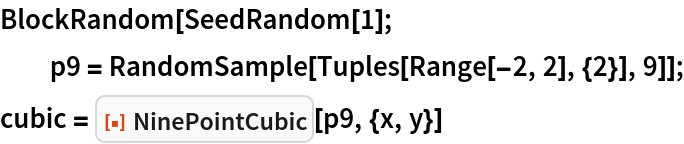Out=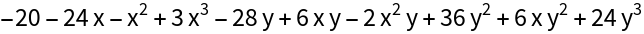Show the cubic and points:

 In:=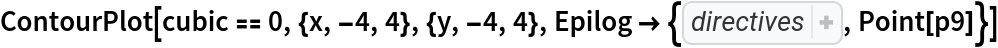Out=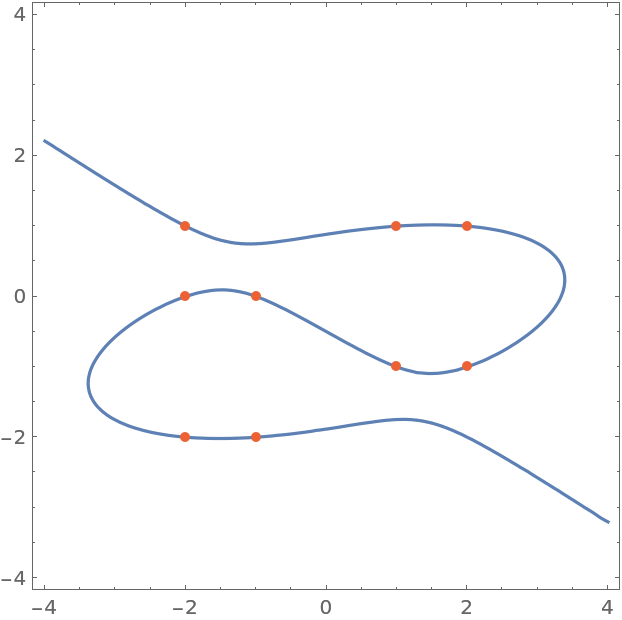### Scope (2)

Find a cubic equation through nine points:

 In:=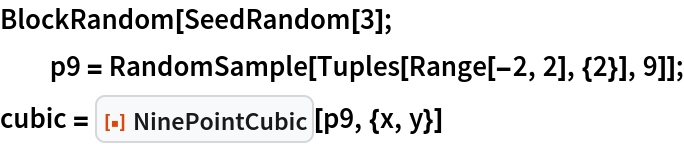Out=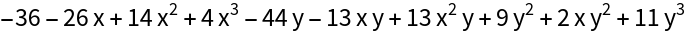Show the cubic and points:

 In:=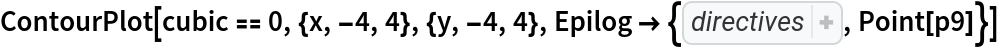Out=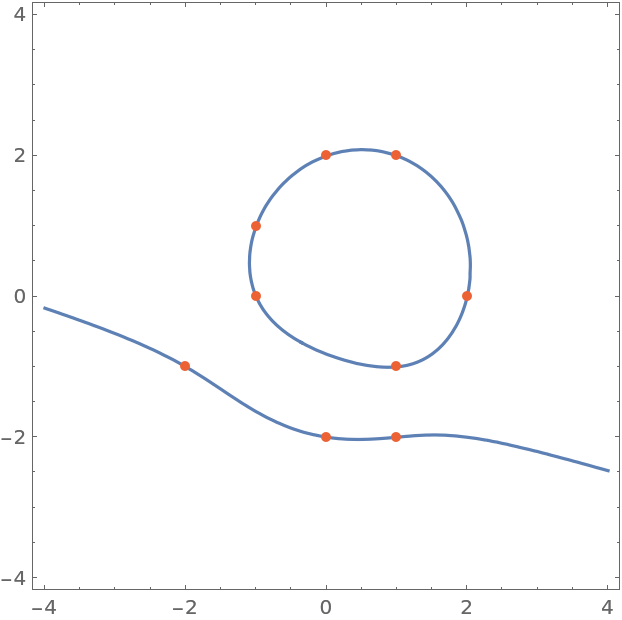Use formal variables:

 In:=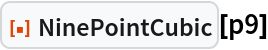Out=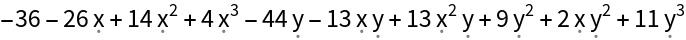Find a cubic through nine points:

 In:=Out=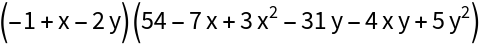The cubic is factorizable and thus degenerate, and is composed of an ellipse and a line:

 In:=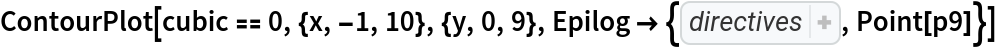Out=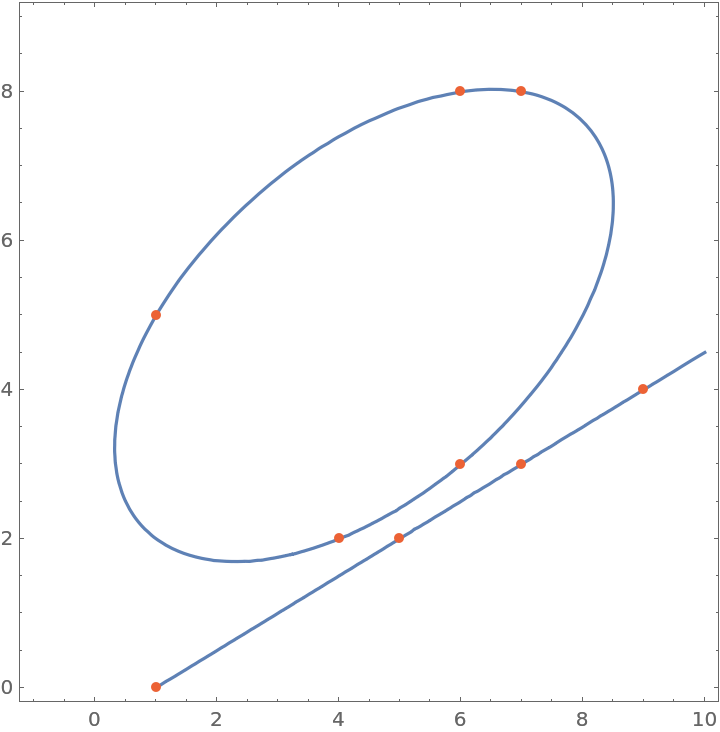### Neat Examples (2)

Some points and a cubic curve:

 In:=Out=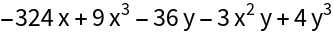Any line going through two points of a cubic will meet one of three criteria: 1. it will go through a third point (gray), 2. it will be tangent at one of the points (red), or 3. it will be parallel with the cubics' asymptote (green), also called a point at infinity:

 In:=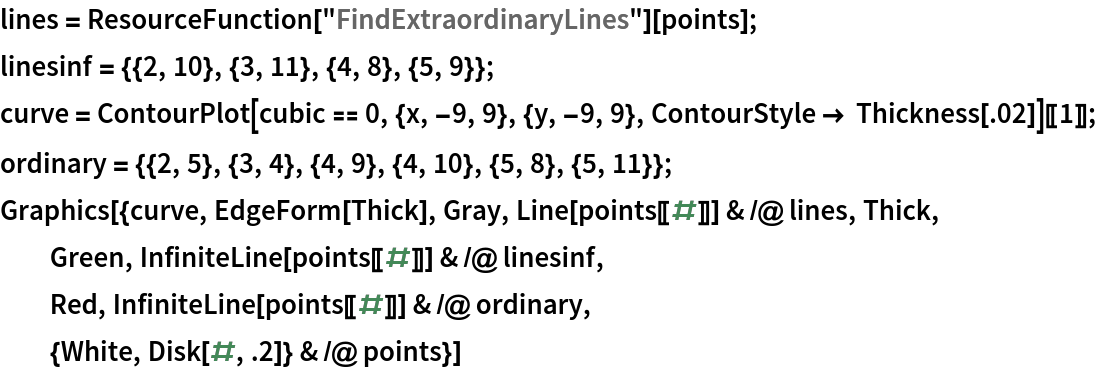Out=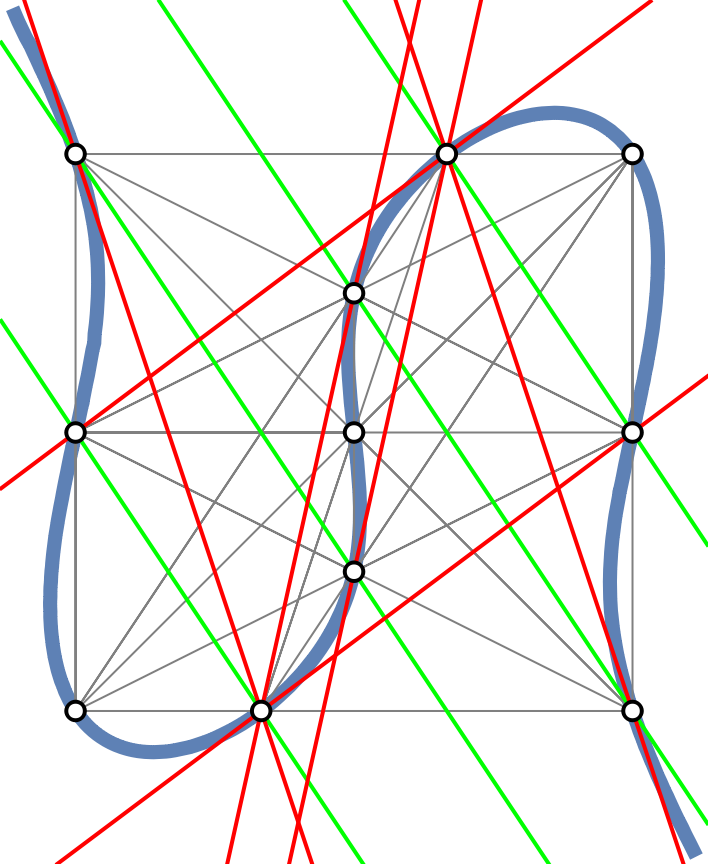## Version History

• 1.0.0 – 26 July 2022